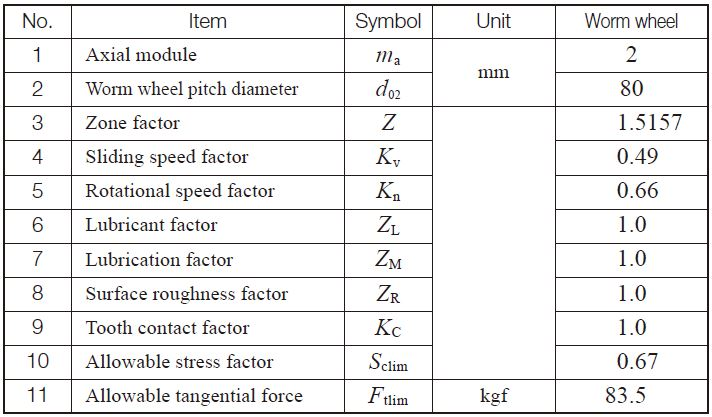TEL : +81 48 254 1744

"Easy" Gear Selection Screen

- Choose a gear by pitch, # of teeth, or bore size.
- 2D/3D Drawings Available
- PDF Catalog Available
- Strength Calculation Available

Go to Gear Selection Screen

CLOSE

# SURFACE DURABILITY OF WORM GEAR

This information is applicable for worm gear pair drives that are used to transmit power in general industrial machines with the following parameters:
Axial module / ma / 1 – 25mm
Pitch diameter of worm wheel / d02 / 900mm or less
Sliding speed / vs / 30m/s or less
Rotational speed of worm wheel / n2 / 600rpm or less

## (1)Basic Formulas

(1)-1 Sliding speed (m/s)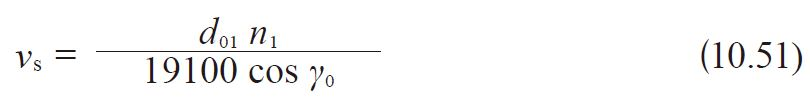(1)-2 Torque, tangential force and efficiency

1. Worm as driver (Speed reducing)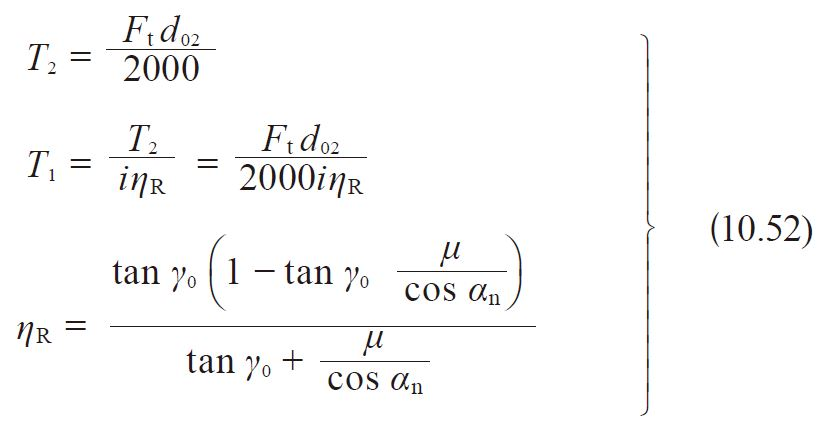Where
T2 ：Nominal torque of worm wheel (kgf・m)
T1 ：Nominal torque of worm (kgf・m)
Ft ：Nominal tangential force on worm wheel’s pitch circle (kgf)
d02 ：Pitch diameter of worm wheel (mm)
i ：Gear ratio = z2 / zw
ηR ：Transmission efficiency, worm driving (not including bearing loss, lubricant agitation loss, etc.)
μ ：Coefficient of friction

2. Worm wheel as driver (Speed increasing)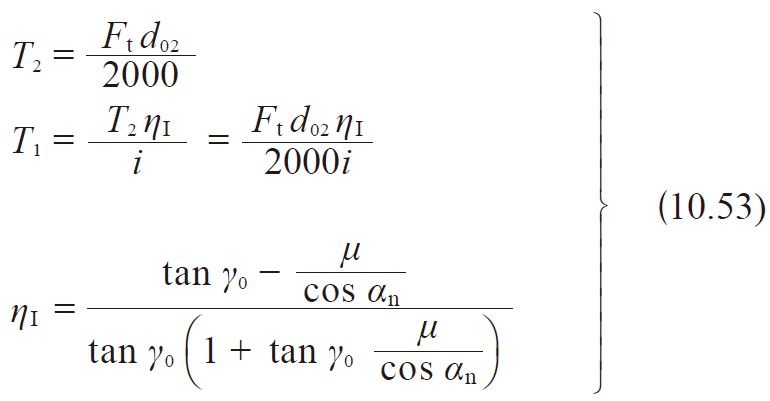Where ηI：Transmission efficiency, worm wheel driving (not including bearing loss, lubricant agitation loss, etc.)

Fig. 10.12 Coefficient of Friction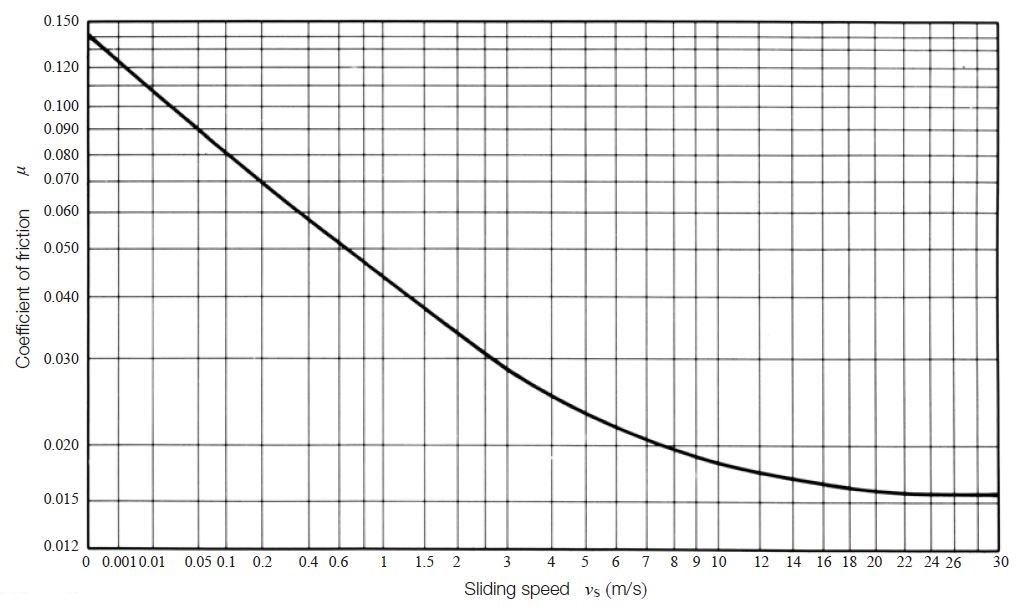For lack of data, coefficient of friction, μ, of materials is very difficult to obtain.
However, Table 10.27 indicates H. E. Merritt’s offer as a reference.

Table 10.27 Combination of materials and their coefficients of friction, μ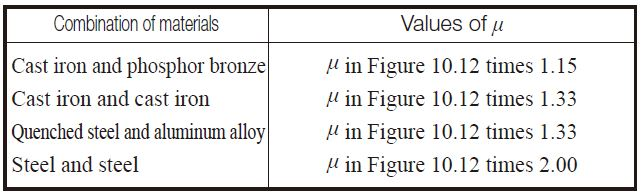3. Coefficient of friction, μ
The coefficient of friction, μ, varies as sliding speed, vS, changes. The combination of materials is important. For the case of a worm that is carburized and ground, and mated with a phosphorous bronze worm wheel, see Figure 10.12.

## (2) Calculation of Allowable Load to Surface Durability

Provided dimensions and materials of the worm gear pair are known, the allowable load is as follows:

Allowable tangential force Ftlim (kgf)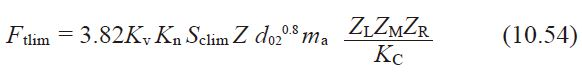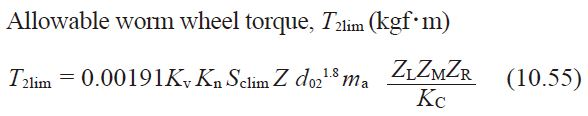Please note that in such cases, where the starting torque is not more than 200% of the rated torque (NOTE 1) and the frequency of starting is less than twice per hour, are regarded as ‘no impact’. In all other cases, the equivalent load is to be calculated and compared to the basic load.
Equivalent load is then converted to an equivalent tangential force, Fte (kgf)

Fte = Ft Kh Ks (10.56)
and equivalent worm wheel torque, T2e (kgf・m)
T2e = T2 Kh Ks (10.57)

[ NOTE 1 ]
Rated torque denotes the torque on the worm wheel when the motor (or loader) is operating at rated load.

1. Under no impact condition, to have life expectancy of 26,000 hours, the following relationships must be satisfied:
Ft Ftlim or T2 T2lim (10.58)
2. For all other conditions:
Fte Ftlim or T2e T2lim (10.59)
NOTE： If load is variable, the comprehensive load, T2C, should be used as the criterion.

## (3) Determination of Factors

(3)-1 Facewidth of Worm Wheel, b2 (mm)
The facewidth of worm wheel, b2, is defined as in Figure 10.13.

Fig. 0.13 Facewidth of worm wheel,b2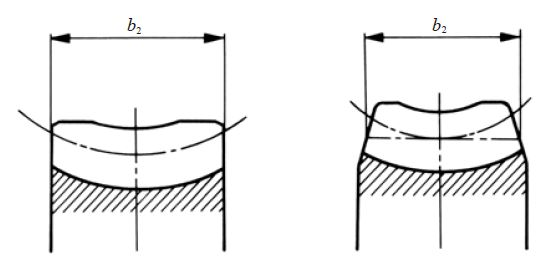(3)-2 Zone factor, Z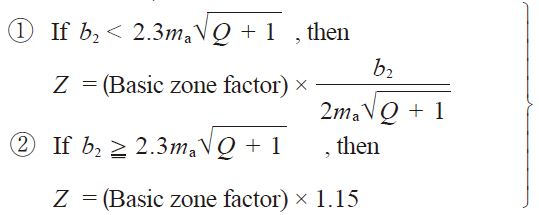(10.60)

Table 10.28 Basic zone factors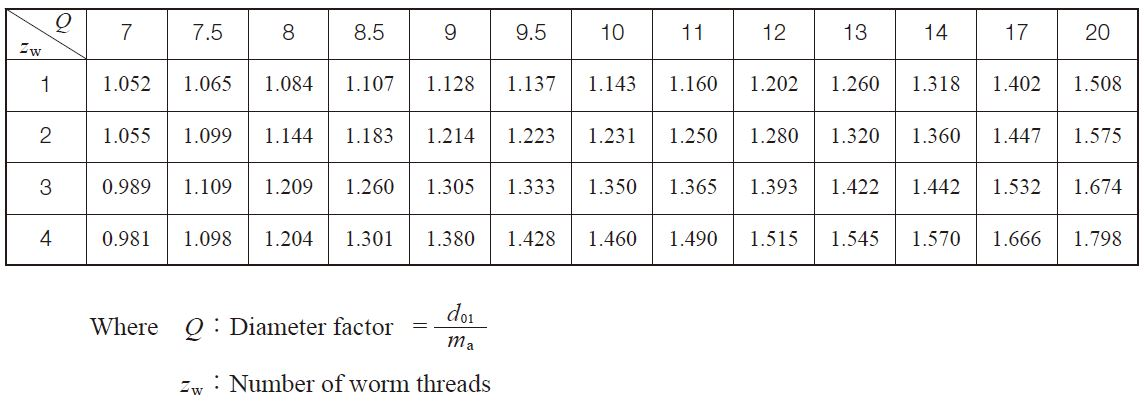(3)-3 Sliding Speed Factor, Kv
Sliding speed factor, Kv, is obtainable from Figure 10.14, where the abscissa is the sliding speed, vs.
Fig. 10.14 Sliding speed factor, Kv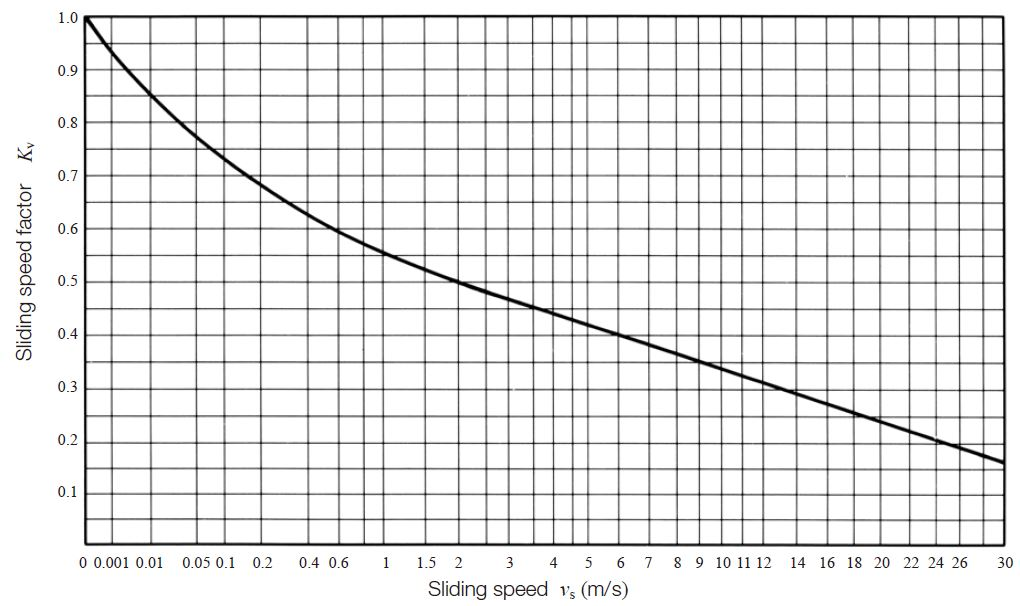(3)-4 Rotational Speed Factor, Kn
The rotational speed factor, Kn, is presented in Figure 10.15 as a function of the worm wheel’s rotational speed, n2 (rpm).

(3)-5 Lubricant Factor, ZL
Let ZL = 1.0 if the lubricant is of proper viscosity and has extreme-pressure additives.
Some bearings in worm gearboxes may need a low viscosity lubricant. Then ZL is to be less than 1.0. The recommended kinetic viscosity of lubrication is given in Table 10.29.

Fig. 10.15 Rotational speed factor, Kn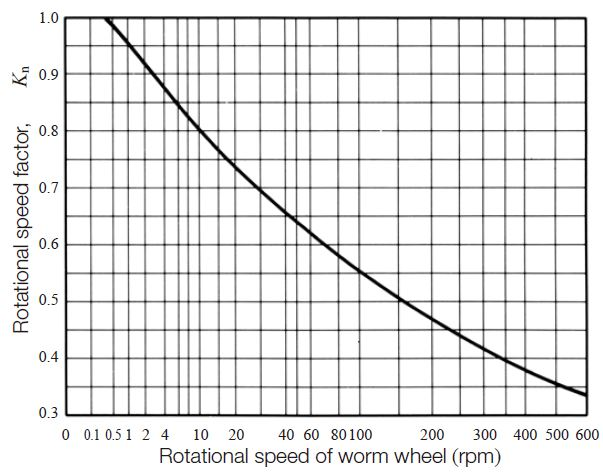Table 10.29 Recommended kinetic viscosity of lubricant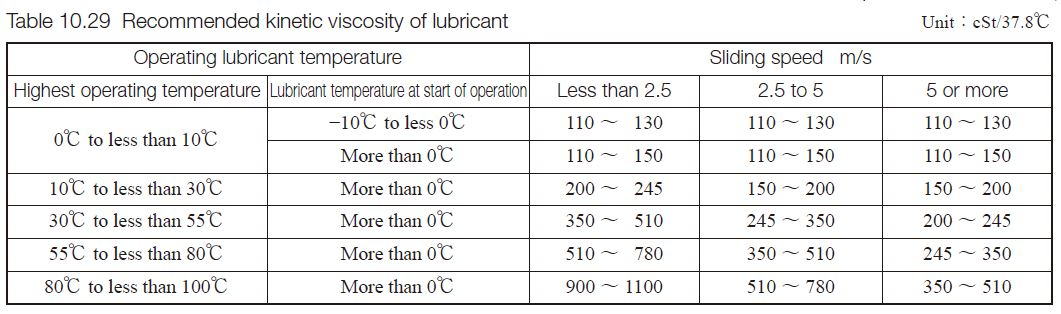(3)-6 Lubrication Factor, ZM
The lubrication factor, ZM, is obtained from Table 10.30.

Table 10.30 Lubrication factor, ZM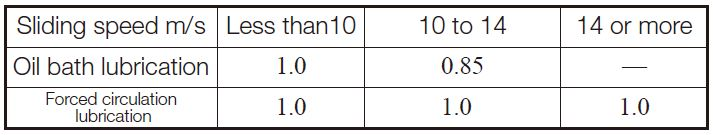(3)-7 Surface Roughness Factor, ZR
The surface roughness factor, ZR, is concerned with resistance to pitting of the working surfaces of the teeth. Since there is insufficient knowledge about this phenomenon, the factor is assumed to be 1.0.
ZR = 1.0 (10.61)
It should be noted that for Equation (10.61) to be applicable, surface roughness of the worm and worm wheel must be less than 3S and 12S respectively. If either is rougher, the factor is to be adjusted to a smaller value.

(3)-8 Tooth Contact Factor, KC
Quality of tooth contact will affect load capacity dramatically. Since it is difficult to prescribe the tooth contact factor, it is usually regarded that KC for Class A of JIS B 1741 is 1.0.
KC = 1.0 (10.62)
For Class B and C, KC should be more than 1.0.
Table 1.31 gives the general values of KC depending on the JIS tooth contact class.

Table 10.31 Classes of tooth contact and general values of tooth contact factor, KC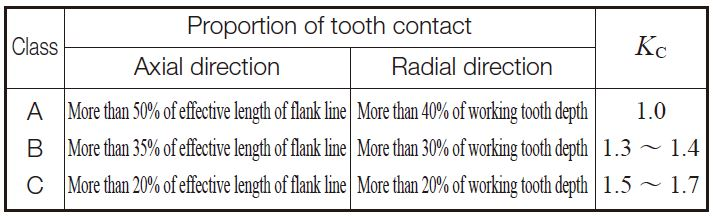(3)-9 Starting Factor, KS
When starting torque is less than 200% of rated torque, KS factor is per Table 10.32.

Table 10.32 Starting factor, KS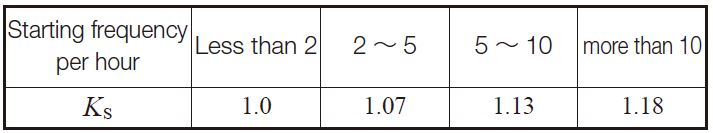(3)-10 Time / Duty Factor, Kh
The time duty factor, Kh, is a function of the desired life and the impact environment. See Table 10.33. The expected lives in between the numbers shown in Table 10.33 can be interpolated.

Table 10.33 Time – duty factor, Kh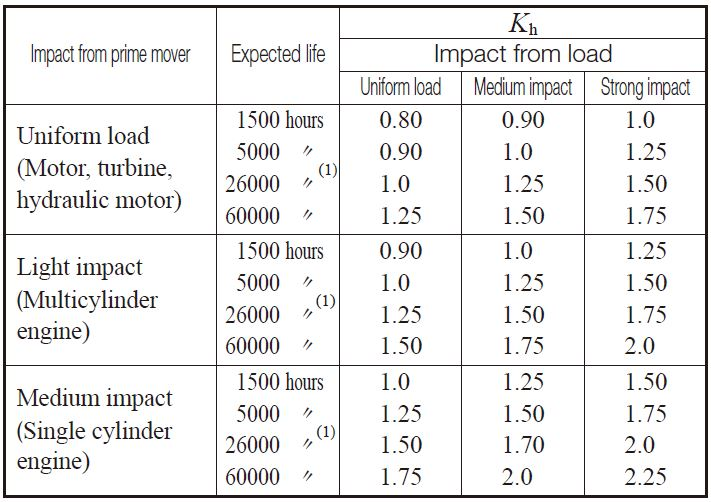NOTE(1) For a machine that operates 10 hours a day, 260 days a year, this number corresponds to ten years of operating life.

(3)-11 Allowable Stress Factor, Sclim
Table 10.34 presents the allowable stress factor, Sclim, for various material combinations. Note that the table also specifies governing limits of sliding speed, which must be adhered to if scoring is to be avoided.

Table 10.34 Allowable stress factor for surface durability, Sclim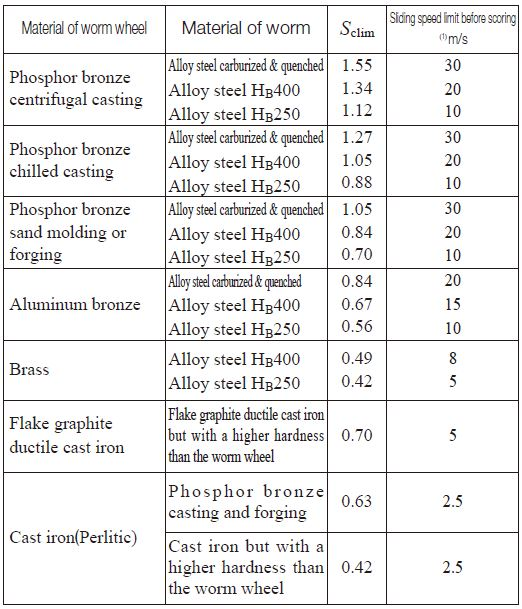NOTE(1) The value indicates the maximum sliding speed within the limit of the allowable stress factor, Sclim. Even when the allowable load is below the allowable stress level, if the sliding speed exceeds the indicated limit, there is danger of scoring gear surfaces.

## (4) Examples of Calculation

Worm gear pair design details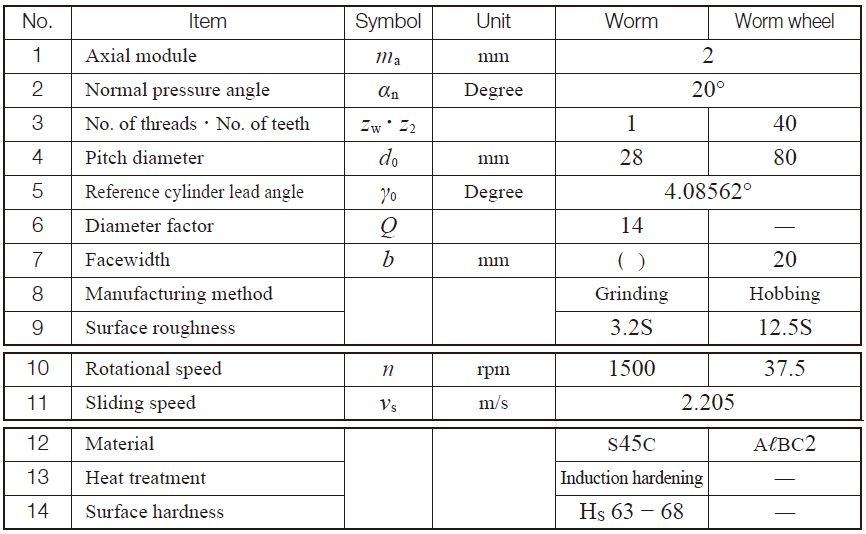Surface durability factors and allowable force